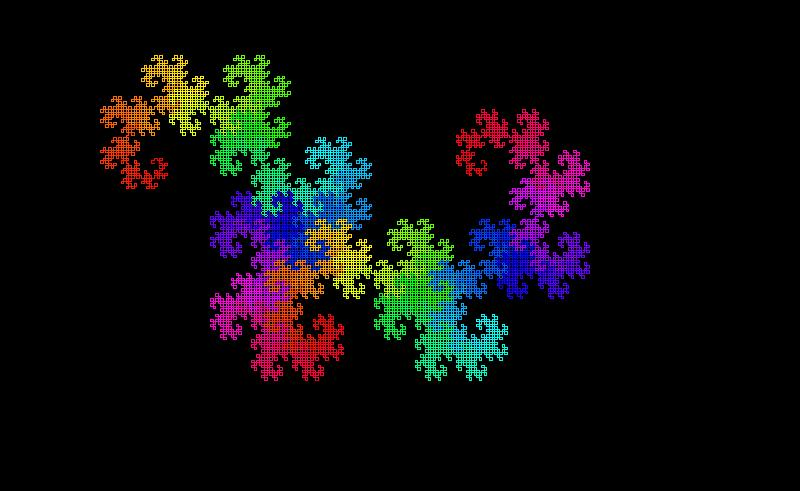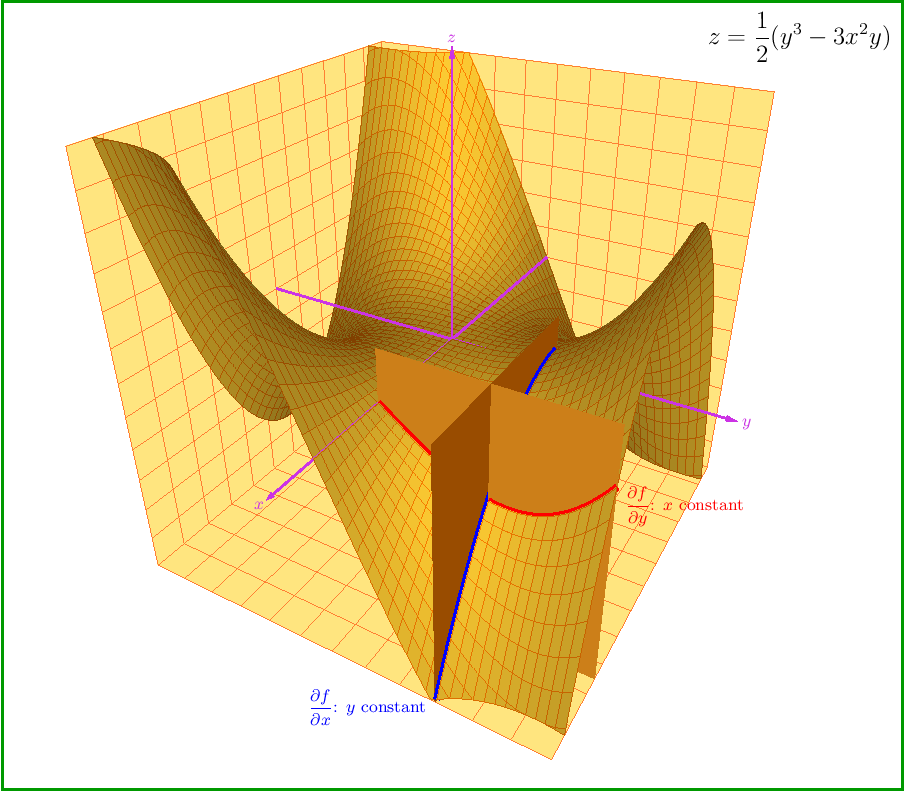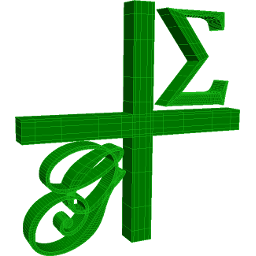## 28 Search Results

### 3D-XplorMath

The primary goal of 3D-XplorMath is to allow users with little or no programming experience to see, with minimal effort, concrete visual representations of many different categories of mathematical objects and processes. To accomplish this, objects from each category are described internally by well-designed, parameterized data structures, and for each category a variety of rendering methods is provided to permit visualization of objects of the category in ways that are appropriate for various purposes. Each of the hundreds of built-in objects known to the program is assigned carefully chosen defaults so that, when the object is selected from a menu, the program can immediately construct a standard example of the object and render it in an optimized view. The user may then use various menus and dialogs to alter the parameters describing the shape and coloration of the object, change the viewpoint from which it is seen, select different rendering methods, etc. Moreover, as its name suggests, the program can display objects such as surfaces, space curves and polyhedra using various stereo techniques. In addition to the many built-in objects known to the program, a user can create "user-defined" objects by entering formulas using standard mathematical notation. Visualizations created by the program can be saved in jpeg and other graphic formats and the data defining 3D objects can be exported to other 3D programs (e.g., Bryce or POV-Ray) in formats such as .obj and .inc. Both built-in and user-defined objects can depend on parameters, and the program can create morphing animations by moving along a path in the parameter space, and these animations can then be saved as QuickTime movies. Each of the built-in objects has associated to it a so-called ATO (About This Object) file that provides documentation for the object. An early and more developed version of the program, written in Object Pascal, runs under the Macintosh Operating System and a Java-based cross-platform version is now also available.

More informationThe package ADOL-C facilitates the evaluation of first and higher derivatives of vector functions that are defined by computer programs written in C or C++. The resulting derivative evaluation routines may be called from C/C++, Fortran, or any other language that can be linked with C. The numerical values of derivative vectors are obtained free of truncation errors at a small multiple of the run time and randomly accessed memory of the given function evaluation program.

### Axiom

Axiom is a general purpose Computer Algebra system. It is useful for research and development of mathematical algorithms. It defines a strongly typed, mathematically correct type hierarchy. It has a programming language and a built-in compiler.

### CoCoA

CoCoA is a system for Computations in Commutative Algebra. It is able to perform simple and sophisticated operations on multivaraiate polynomials and on various data related to them (ideals, modules, matrices, rational functions). For example, it can readily compute Grobner bases, syzygies and minimal free resolution, intersection, division, the radical of an ideal, the ideal of zero-dimensional schemes, Poincare' series and Hilbert functions, factorization of polynomials, toric ideals. The capabilities of CoCoA and the flexibility of its use are further enhanced by the dedicated high-level programming language. For convenience, the system offers a textual interface, an Emacs mode, and a graphical user interface common to most platforms.

### ePix

ePiX, a collection of batch-oriented utilities for *nix, creates mathematically accurate line figures, plots, and movies using easy-to-learn syntax. LaTeX and dvips comprise the typographical rendering engine, while ImageMagick is used to create bitmapped images and animations. The user interface resembles that of LaTeX itself: You prepare a short scene description in a text editor, then compile'' the input file into a picture. Default output formats are eepic (a plain text enhancement to the LaTeX picture environment), eps, pdf, png, and mng.

More information### G+Smo

G+Smo (Geometry + Simulation Modules, pronounced "gismo") is a new open-source C++ library that brings together mathematical tools for geometric design and numerical simulation. It is developed mainly by researchers and PhD students. It implements the relatively new paradigm of isogeometric analysis, which suggests the use of a unified framework in the design and analysis pipeline. G+Smo is an object-oriented, cross-platform, template C++ library and follows the generic programming principle, with a focus on both efficiency and ease of use. The library is partitioned into smaller entities, called modules. Examples of available modules include the dimension-independent NURBS module, the data fitting and solid segmentation module, the PDE discretization module and the adaptive spline module, based on hierarchical splines of arbitrary dimension and polynomial degree. The library is licenced under the Mozilla Public License v2.0. It has been developed within the homonym research network supported by the Austrian Science Fund and aims at providing access to high quality, open-source software to the forming isogeometric numerical simulation community and beyond.

More information### GELDA

GELDA is a Fortran77 software package for the numerical integration of general linear differential-algebraic equations (DAE) with variable coefficients of arbitrary index. The implementation of GELDA is based on the construction of the discretization scheme, which first determines all the local invariants and then transforms the linear DAE into an equivalent strangeness-free DAE with the same solution set. The resulting strangeness-free system is allowed to have nonuniqueness in the solution set or inconsistency in the initial values or inhomogeneities. In the case that the DAE is found to be uniquely solvable, GELDA is able to compute a consistent initial value and apply the well-known integration schemes for DAEs. In GELDA the BDF methods and Runge-Kutta schemes are implemented.

### GEOMS

GEOMS is a Fortran77 software package for the numerical integration of general model equations (equations of motion) of multibody systems. The code combines a regularization technique with the Runge-Kutta method of type Radau IIa of order 5. This regularization technique reduces the index under preservation of all information of the solution manifold and, therefore, allows a robust numerical integration of the system.

### HiFlow³

HiFlow³ is a multi-purpose finite element software providing powerful tools for efficient and accurate solution of a wide range of problems modeled by partial differential equations. Based on object-oriented concepts and the full capabilities of C++ the HiFlow³ project follows a modular and generic approach for building efficient parallel numerical solvers. It provides highly capable modules dealing with the mesh setup, finite element spaces, degrees of freedom, linear algebra routines, numerical solvers, and output data for visualization. Parallelism – as the basis for high performance simulations on modern computing systems – is introduced on two levels: coarse-grained parallelism by means of distributed grids and distributed data structures, and fine-grained parallelism by means of platform-optimized linear algebra back-ends.

### HSL

HSL (formerly the Harwell Subroutine Library) is a collection of ISO Fortran codes for large scale scientific computation, written by members of the Numerical Analysis Group and other experts.

### ISAAC

ISAAC (Integrated Solution Algorithm for Arbitrary Configurations) is a compressible Euler/Navier-Stokes computational fluid dynamics code. ISAAC includes the capability of calculating the Euler equations for inviscid flow or the Navier-Stokes equations for viscous flows. ISAAC uses a domain decomposition structure to accomodate complex physical configurations. ISAAC can calculate either steady-state or time dependent flow. ISAAC was designed to test turbulence models. Various two equation turbulence models, explicit algebraic Reynolds stress models, and full differential Reynolds stress models are implemented in ISAAC. Several test cases are documented in the User's Guide.

### KANT

KASH/KANT is a computer algebra system for sophisticated computations in algebraic number fields and global function fields. It has been developed under the project leadership of Prof. Dr. M. Pohst at Technische Universität Berlin.

### Magma

Magma is a large, well-supported software package designed to solve computationally hard problems in algebra, number theory, geometry and combinatorics. It provides a mathematically rigorous environment for computing with algebraic, number-theoretic, combinatoric and geometric objects.

### Maple

Maple is an environment for scientific and engineering problem-solving, mathematical exploration, data visualization and technical authoring.

More information### Mathematica

Mathematica seamlessly integrates a numeric and symbolic computational engine, graphics system, programming language, documentation system, and advanced connectivity to other applications.

### Matlab

MATLAB is a high-level language and interactive environment that enables you to perform computationally intensive tasks faster than with traditional programming languages such as C, C++, and Fortran.

### Maxima

Maxima is a system for the manipulation of symbolic and numerical expressions, including differentiation, integration, Taylor series, Laplace transforms, ordinary differential equations, systems of linear equations, and vectors, matrices, and tensors. Maxima produces high precision results by using exact fractions and arbitrarily long floating point representations, and can plot functions and data in two and three dimensions.

MuPAD is a mathematical expert system for doing symbolic and exact algebraic computations as well as numerical calculations with almost arbitrary accuracy. For example, the number of significant digits can be chosen freely. Apart from a vast variety of mathematical libraries the system provides tools for high quality visualization of 2- and 3-dimensional objects. On Microsoft Windows, Apple Macintosh and Linux systems, MuPAD offers a flexible notebook concept for creating mathematical documents combining texts, graphics, formulas, computations and mathematical visualizations and animations. On Microsoft Windows MuPAD further supports the technologies OLE, ActiveX Automation, DCOM, RTF and HTML. Thus it offers a natural integration in Office applications like Word or PowerPoint as well as others.

### Octave

GNU Octave is a high-level language, primarily intended for numerical computations. It provides a convenient command line interface for solving linear and nonlinear problems numerically, and for performing other numerical experiments using a language that is mostly compatible with Matlab. It may also be used as a batch-oriented language. Octave has extensive tools for solving common numerical linear algebra problems, finding the roots of nonlinear equations, integrating ordinary functions, manipulating polynomials, and integrating ordinary differential and differential-algebraic equations. It is easily extensible and customizable via user-defined functions written in Octave's own language, or using dynamically loaded modules written in C++, C, Fortran, or other languages.

### PLTMG

PLTMG is a package for solving elliptic partial differential equations in general regions of the plane. It is based on continuous piecewise linear triangular finite elements, and features adaptive local mesh refinement, multigraph iteration, and pseudo-arclength continuation options for parameter dependencies. It also provides options for solving several classes of optimal control and obstacle problems. The package includes an initial mesh generator and several graphics packages. Support for the Bank-Holst parallel adaptive meshing strategy is also provided. PLTMG is provided as Fortran (and a little C) source code, in both single and double precision versions. The code has interfaces to X-Windows, MPI, and Michael Holst's OpenGL image viewer SG. The X-Windows, MPI, and SG interfaces require libraries that are NOT provided as part of the PLTMG package.

### rbMIT

The rbMIT © MIT software package implements in Matlab® all the general reduced basis algorithms. The rbMIT © MIT software package is intended to serve both (as Matlab® source) "Developers" — numerical analysts and computational tool-builders — who wish to further develop the methodology, and (as Matlab® "executables") "Users" — computational engineers and educators — who wish to rapidly apply the methodology to new applications. The rbMIT software package was awarded with the Springer Computational Science and Engineering Prize in 2009.

### Reduce

REDUCE is an interactive system for general algebraic computations of interest to mathematicians, scientists and engineers. It has been produced by a collaborative effort involving many contributors. It is often used as an algebraic calculator for problems that are possible to do by hand. However, REDUCE is designed to support calculations that are not feasible by hand. Many such calculations take a significant time to set up and can run for minutes, hours or even days on the most powerful computers.

### Risa/Asir

Risa/Asir is a general computer algebra system and also a tool for various computation in mathematics and engineering. The development of Risa/Asir started in 1989 at FUJITSU. Binaries have been freely available since 1994 and now the source code is also free. Currently Kobe distribution is the most active branch of its development. We characterize Risa/Asir as follows: (1) An environment for large scale and efficient polynomial computation. (2) A platform for parallel and distributed computation based on OpenXM protocols.

### Sage

SAGE is a framework for number theory, algebra, and geometry computation. It is open source and freely available under the terms of the GNU General Public License (GPL). SAGE is a Python library with a customized interpreter. It is written in Python, C++, and C (via Pyrex). Python (http://www.python.org) is an open source object-oriented interpreted language, with a large number of libraries, e.g., for numerical analysis, which are available to users of SAGE. Python can also be accessed in library mode from C/C++ programs. SAGE provides an interface to several important open source libraries, including Cremona’s MWRANK library for computing with elliptic curves, the PARI library (pari.math.u-bordeaux.fr) for number theory, Shoup’s number theory library NTL (http://www.shoup.net/ntl/), SINGULAR (http://www.singular.uni-kl.de) for commutative algebra, GAP (http://www.gap-system.org) for group theory and combinatorics, and maxima (http://maxima.sourceforge.net) for symbolic computation and calculus.

More information### Scilab

Scilab is a numerical computation system similiar to Matlab or Simulink. Scilab includes hundreds of mathematical functions, and programs from various languages (such as C or Fortran) can be added interactively. It has sophisticated data structures (including lists, polynomials, rational functions, and linear systems), an interpreter, and a high-level programming language. Scilab has been designed to be an open system where the user can define new data types and operations on these data types by using overloading. A number of toolboxes are available with the system.

### SingSurf

An online Java applet for calculation of singular algebraic surfaces.